true
true

Ganesh WadkarDhanori, Pune, India - 411015# Ganesh Wadkar## Tutor

Dhanori, Pune, India - 411015.

2 Students taught

5.0

UrbanPro Rating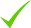IdentityEducation

Know how UrbanPro verifies Tutor details

Identity is verified based on matching the details uploaded by the Tutor with government databases.

## Overview

I am an experienced, qualified tutor with over 5 years of experience in teaching physics and maths.passionate about teaching physics concepts and maths numericals. I have good experience of competitive examinations like NDA, CDSE.

## Languages Spoken

Marathi Mother Tongue (Native)

English Proficient

Hindi Proficient

## Education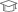fergusson 2013

Bachelor of Science (B.Sc.)pune university 2015

Master of Science (M.Sc.)

Dhanori, Pune, India - 411015

## Verified Info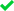ID VerifiedEducation VerifiedPhone VerifiedEmail Verified

## Demo Class

Yes, charged

Report this Profile

Is this listing inaccurate or duplicate? Any other problem?

Type the letters as shown below *

Please enter the letters as show below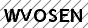## Class 8 Tuition Overview

Class 8 Tuition

Class LocationStudent's HomeTutor's Home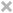Online (video chat via skype, google hangout etc)

Years of Experience in Class 8 Tuition

4

Board

State, ICSE, CBSE

CBSE Subjects taught

Mathematics, Science

ICSE Subjects taught

Physics

Experience in School or College

I have done my internship from reputed school and it was good experience. I am associated with coaching institute where I am teaching Physics for 11th and 12th std from last 4 years.

Taught in School or College

Yes

State Syllabus Subjects taught

Science, Mathematics

## Reviews

this is test message this is test message this is test message this is test message this is test message this is test message this is test message

No Reviews yet! Be the first one to Review

## FAQs

1. Which school boards of of Class 8 do you teach for?

State, ICSE and CBSE

2. Have you ever taught in any School or College?

Yes

3. Which classes do you teach?

I teach Class 10 Tuition, Class 11 Tuition, Class 12 Tuition, Class 7 Tuition, Class 8 Tuition, Class 9 Tuition and UPSC Exams Coaching Classes.

4. Do you provide a demo class?

Yes, I provide a paid demo class.

5. How many years of experience do you have?

I have been teaching for 4 years.

You can guess the square root with simple logic. For example 81. - sq root is 9 361 is sq root of number whose unit = 1 or 9 Square of 20 is 400 .so u can it's square root of either 11 or 19. If you compare sq of 20 u can easily guess it sq root of 19. If last digit is 4 then it's square root of... ...more

You can guess the square root with simple logic.

For example

81.   - sq root is 9

361 is sq root of number whose unit = 1 or 9

Square of 20 is 400 .so u can it's square root of either 11 or 19.

If you compare sq of 20 u can easily guess it sq root of 19.

If last digit is 4 then it's square root of either 2 or 8

If last digit is 9 then it's square root of either 7 or 3

If last digit is 6 then it's square root of either 4 or 6

And if it's 5 then 5

Dislike Bookmark

Answered on 18/08/2019 CBSE/Class 9/Mathematics/Surface Area and Volumes/NCERT Solutions/Exercise 13.7

given h = 15 cm volume of cone = 1570 to find- radius (r) formula- volume of cone = 1570 = 3.14 * * 15 /3 1570 *3 = 3.14 * 15 * = 1570 * 3 / 3.14 * 15 =100 taking square roots on both sides , we get r = 10 cm ...more

given

h = 15 cm

volume of cone = 1570

formula-

volume of cone =

1570     = 3.14 *  * 15 /3

1570 *3 =  3.14 * 15 *

=   1570 * 3 / 3.14 * 15

=100

taking square roots on both sides , we get

r = 10 cm

Dislike Bookmark

Answered on 18/08/2019 CBSE/Class 9/Mathematics/Surface Area and Volumes/NCERT Solutions/Exercise 13.7

Diameter of the conical pit = 3.5m radius= 1.75m height=12m volume of cone= π h /3 = 3.14 * 1.75^2 * 12 /3 =115.39/3 = 38.46 volume of cone = 38.46 m^3 1= 1000 L = 1 kilolitre hence , 38.5 = 38.5 kilolitrs ...more

Diameter of the conical pit = 3.5m

height=12m

volume of cone= π h /3

= 3.14 * 1.75^2 * 12  /3

=115.39/3

= 38.46

volume of cone = 38.46 m^3

1= 1000 L = 1 kilolitre

hence ,

38.5  = 38.5 kilolitrs

Dislike Bookmark

Answered on 17/08/2019 CBSE/Class 9/Mathematics/Surface Area and Volumes/NCERT Solutions/Exercise 13.7

1. L^2 = h^2 + r^2 L = slant height h= height r= radius 25^2 = h^2 + 7^2 h^2 = 625 - 49 = 576 after taking square root h = 24 volume of cone = L^2 = h^2 + r^2 = 1/3 * 3.14 *7^2 *... ...more

1.    L^2 = h^2 + r^2              L = slant height   h= height  r= radius

25^2 = h^2 + 7^2

h^2 = 625 - 49 = 576

after taking square root

h = 24

volume of cone =                               L^2 = h^2 + r^2

= 1/3 * 3.14 *7^2 * 24

=3692.64/3

volume of cone  =1230 cm^3

1L = 1000cm^3

Dislike Bookmark
Class 8 Tuition 5.0

Class LocationStudent's HomeTutor's HomeOnline (video chat via skype, google hangout etc)

Years of Experience in Class 8 Tuition

4

Board

State, ICSE, CBSE

CBSE Subjects taught

Mathematics, Science

ICSE Subjects taught

Physics

Experience in School or College

I have done my internship from reputed school and it was good experience. I am associated with coaching institute where I am teaching Physics for 11th and 12th std from last 4 years.

Taught in School or College

Yes

State Syllabus Subjects taught

Science, Mathematics

Class 9 Tuition 5.0

Class LocationStudent's HomeTutor's HomeOnline (video chat via skype, google hangout etc)

Years of Experience in Class 9 Tuition

4

Board

CBSE, ICSE, State

CBSE Subjects taught

Mathematics, Science

ICSE Subjects taught

Physics

Taught in School or College

No

State Syllabus Subjects taught

Science, Mathematics

Class 10 Tuition 5.0

Class LocationStudent's HomeTutor's HomeOnline (video chat via skype, google hangout etc)

Years of Experience in Class 10 Tuition

4

Board

CBSE, ICSE, State

CBSE Subjects taught

Mathematics, Science

ICSE Subjects taught

Physics

Taught in School or College

No

State Syllabus Subjects taught

Science, Mathematics

Class 7 Tuition 5.0

Class LocationStudent's HomeTutor's HomeOnline (video chat via skype, google hangout etc)

Years of Experience in Class 7 Tuition

4

Board

State, ICSE, CBSE

CBSE Subjects taught

Mathematics

ICSE Subjects taught

Physics

Taught in School or College

No

State Syllabus Subjects taught

Science, Mathematics

Class 11 Tuition 5.0

Class LocationStudent's HomeTutor's HomeOnline (video chat via skype, google hangout etc)

Years of Experience in Class 11 Tuition

5

Board

CBSE, ISC/ICSE, State

ISC/ICSE Subjects taught

Physics, Mathematics

CBSE Subjects taught

Mathematics, Physics

Taught in School or College

No

State Syllabus Subjects taught

Physics, History, Mathematics, Geography

Class 12 Tuition 5.0

Class LocationStudent's HomeTutor's HomeOnline (video chat via skype, google hangout etc)

Years of Experience in Class 12 Tuition

4

Board

CBSE, ISC/ICSE, State

ISC/ICSE Subjects taught

Physics

CBSE Subjects taught

Physics

Taught in School or College

No

State Syllabus Subjects taught

Physics, Mathematics

UPSC Exams Coaching classes 5.0

Class LocationStudent's HomeTutor's HomeOnline (video chat via skype, google hangout etc)

Years of Experience in UPSC Exams Coaching classes

4

Gives

NDA (National Defence Acadamy and Naval Acadamy) Exam Coaching, CDS (Combined Defence Service) Exam Coaching

this is test message this is test message this is test message this is test message this is test message this is test message this is test message

No Reviews yet! Be the first one to Review

You can guess the square root with simple logic. For example 81. - sq root is 9 361 is sq root of number whose unit = 1 or 9 Square of 20 is 400 .so u can it's square root of either 11 or 19. If you compare sq of 20 u can easily guess it sq root of 19. If last digit is 4 then it's square root of... ...more

You can guess the square root with simple logic.

For example

81.   - sq root is 9

361 is sq root of number whose unit = 1 or 9

Square of 20 is 400 .so u can it's square root of either 11 or 19.

If you compare sq of 20 u can easily guess it sq root of 19.

If last digit is 4 then it's square root of either 2 or 8

If last digit is 9 then it's square root of either 7 or 3

If last digit is 6 then it's square root of either 4 or 6

And if it's 5 then 5

Dislike Bookmark

Answered on 18/08/2019 CBSE/Class 9/Mathematics/Surface Area and Volumes/NCERT Solutions/Exercise 13.7

given h = 15 cm volume of cone = 1570 to find- radius (r) formula- volume of cone = 1570 = 3.14 * * 15 /3 1570 *3 = 3.14 * 15 * = 1570 * 3 / 3.14 * 15 =100 taking square roots on both sides , we get r = 10 cm ...more

given

h = 15 cm

volume of cone = 1570

formula-

volume of cone =

1570     = 3.14 *  * 15 /3

1570 *3 =  3.14 * 15 *

=   1570 * 3 / 3.14 * 15

=100

taking square roots on both sides , we get

r = 10 cm

Dislike Bookmark

Answered on 18/08/2019 CBSE/Class 9/Mathematics/Surface Area and Volumes/NCERT Solutions/Exercise 13.7

Diameter of the conical pit = 3.5m radius= 1.75m height=12m volume of cone= π h /3 = 3.14 * 1.75^2 * 12 /3 =115.39/3 = 38.46 volume of cone = 38.46 m^3 1= 1000 L = 1 kilolitre hence , 38.5 = 38.5 kilolitrs ...more

Diameter of the conical pit = 3.5m

height=12m

volume of cone= π h /3

= 3.14 * 1.75^2 * 12  /3

=115.39/3

= 38.46

volume of cone = 38.46 m^3

1= 1000 L = 1 kilolitre

hence ,

38.5  = 38.5 kilolitrs

Dislike Bookmark

Answered on 17/08/2019 CBSE/Class 9/Mathematics/Surface Area and Volumes/NCERT Solutions/Exercise 13.7

1. L^2 = h^2 + r^2 L = slant height h= height r= radius 25^2 = h^2 + 7^2 h^2 = 625 - 49 = 576 after taking square root h = 24 volume of cone = L^2 = h^2 + r^2 = 1/3 * 3.14 *7^2 *... ...more

1.    L^2 = h^2 + r^2              L = slant height   h= height  r= radius

25^2 = h^2 + 7^2

h^2 = 625 - 49 = 576

after taking square root

h = 24

volume of cone =                               L^2 = h^2 + r^2

= 1/3 * 3.14 *7^2 * 24

=3692.64/3

volume of cone  =1230 cm^3

1L = 1000cm^3

Dislike Bookmark

Ganesh Wadkar describes himself as Tutor. He conducts classes in Class 10 Tuition, Class 11 Tuition and Class 12 Tuition. Ganesh is located in Dhanori, Pune. Ganesh takes at students Home and Regular Classes- at his Home. He has 5 years of teaching experience . Ganesh has completed Bachelor of Science (B.Sc.) from fergusson in 2013 and Master of Science (M.Sc.) from pune university in 2015. He is well versed in English, Hindi and Marathi.

Recommended Profiles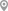Jadavpur, KolkataAttapur, HyderabadNanded, PuneDhanori, PunePatel Nagar, DelhiManik Baug, Pune

X

Let us shortlist and give the best tutors and institutes.

or

Let Ganesh Wadkar know you are interested in their class